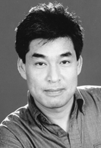Mathematical Models and a Theory of Biological Population Dynamics: From Cells to Ecology, Small and Large Systems
Speaker
Prof. Hong Qian
University of Washington, USA
Abstract

Complex dynamics of interacting populations of intrinsically stochastic individuals can be mathematically represented by a discrete-state, continuous-time Markov jump process.  T. G. Kurtz's theorem establishes a relation between this stochastic process, in the limit of V tending infinity, and the traditional dynamical systems based on ODEs.  We apply this theory to several problems in current cell biology in terms of the biochemical constituents, and illustrate the emergent notions of epigenetic phenotypes and their switching, and relation to the classical idea of phase transition.  The mathematical origin of the latter in non-uniform convergence when time goes to infinity with respect to the parameter V is discussed, and a large deviations result based on WKB ansatz will be presented. We suggest some open questions and a set of computational issues as well.